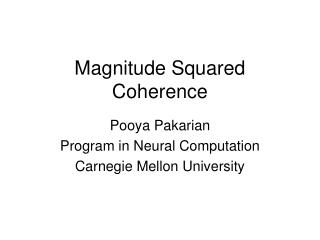DownloadDownload PresentationMagnitude Squared Coherence

# Magnitude Squared Coherence

Télécharger la présentation## Magnitude Squared Coherence

- - - - - - - - - - - - - - - - - - - - - - - - - - - E N D - - - - - - - - - - - - - - - - - - - - - - - - - - -
##### Presentation Transcript

1. Magnitude Squared Coherence Pooya Pakarian Program in Neural Computation Carnegie Mellon University

2. Magnitude Squared Coherence is the cosine squared of the angle between two vectors. Each vector represents the Fourier transform of a given frequency across several epochs of a time series signal. As is the output of Fourier transform, the elements of these vectors are complex numbers. Calculating the Magnitude Squared Coherence is like calculating the correlation coefficient squared between two vectors of zero-mean stationary complex time series.

3. Coherence analysis between two signals

4. 3 seconds Cos(-60°)=0.5 5 Hz, magnitude=1 volt, initial phase=-60° (w/r/t a cosine wave)

5. Both amplitude and phase modulation, the negative multiplier adds (or subtracts) 180° to (from) the phase. magnitude modulation

6. Step 1: cut the signals to several snippets, conduct DFT on each. magn=1 volt phase=-60° magn=1 volt phase=-60° magn=1 volt phase=-60° DFT of 5 Hz: magn=2.48 volt phase=-60° magn=4.02 volt phase=-60° magn=2 volt phase=+120° Hint: the lower signal does have non-zero energy in other frequencies as well, but in this example we are only studying its 5 Hz component. Hint: I chose the phase modulation in the lower signal to occur at the start of its third snippet, but this is not necessarily the case in real world practice.

7. Two complex vectors represent the DFT of 5 Hz of the two signals Signal #1 Signal #2 An arbitrary example of maximum coherence (=1) Signal #1 Signal #2 A consistent divisive relation between the magnitudes and a consistent subtractive relation between the phases

8. Step 2: the numerator of coherence: inner product of the two complex vectors each element of vector 1 multiplied in the conjugate of their corresponding element in signal 2 magnitudes multiplied Phases subtracted Signal #1 Signal #2 The subtractive effect of the phase: The magnitudes are always positive, so are their products. The inequality of the phase difference, but, may force them to be subtracted from each other so that even the numerator of coherence becomes zero. the numerator of coherence

9. Step 3: the denominator of coherence: the product of the sizes of complex vectors Signal #1 Signal #2 The divisive effect of the magnitude: Assume that all the phase differences are equal to zero, so in the numerator we would have the inner product of two all positive vectors, that is always less than the denominator calculated here or equal to it, but can never be zero.

10. Step 4: (magnitude squared) coherence= numerator times its conjugate divided by the square of the product of the sizes = 0.5065 2 Note the conjugation of phase in the numerator. In this particular example that the phase happened to be zero, its effect is not much apparent, but if the it is very helpful by forcing the result to be a real number with zero imaginary part because the phase would be subtracted from itself, and thus the result would be equal to the magnitude squared.

11. An example from the literature Wang S, Aziz TZ, Stein JF, Bain PG, Liu X. 2006 Jul;117(7):1487-98. Clin Neurophysiol. Physiological and harmonic components in neural and muscular coherence in Parkinsonian tremor. As you see, the two signals are divided into “nd” segments. The key point is that, in the numerator, the cross spectrum for each single frequency (represented byfabove) is first summed across segments and then its modulus is calculated and raised to the power of two. In the denominator, in contrast, the complex numbers of auto-spectrum are first subject to calculation of their modulus and being squared, and then the summation is performed. These are complex numbers, therefore, the modulus of their sum can easily be much less than the sum of their modulus, i.e. no surprise that the numerator becomes less than the denominator, leading into a coherence less than “1”. Note that the segment-wise multiplication and then summation in the numerator is like the inner product of two multidimensional complex vectors.. Minor point: the modulus function in the denominator was not necessary, because the power of two is implemented by multiplication of a complex number by its own conjugate that anyhow zeros out the phase and simply raises the magnitude to the power of two.

12. Further readings (the same method) Srinivasan R, Winter WR, Ding J, Nunez PL. EEG and MEG coherence: measures of functional connectivity at distinct spatial scales of neocortical dynamics. J Neurosci Methods. 2007 Oct 15;166(1):41-52. Pfurtscheller G, Andrew C Event-Related changes of band power and coherence: methodology and interpretation. J Clin Neurophysiol. 1999 Nov;16(6):512-9. Farmer SF, Bremner FD, Halliday DM, Rosenberg JR, Stephens JA. The frequency content of common synaptic inputs to motoneurones studied during voluntary isometric contraction in man. J Physiol. 1993 Oct;470:127-55. (This article, among others, cites Bloomfield 1976 for its method and also mentions, as is also implied from the present series of slides, that smoothing of the spectrograms during the process of coherence analysis is simply to reduce the high frequency noise, it is not a genuine part of coherence analysis). Halliday DM, Rosenberg JR, Amjad AM, Breeze P, Conway BA, Farmer SF. A framework for the analysis of mixed time series/point process data--theory and application to the study of physiological tremor, single motor unit discharges and electromyograms. Prog Biophys Mol Biol. 1995;64(2-3):237-78. Coherence and other analyses in the mixed time series/point process data!